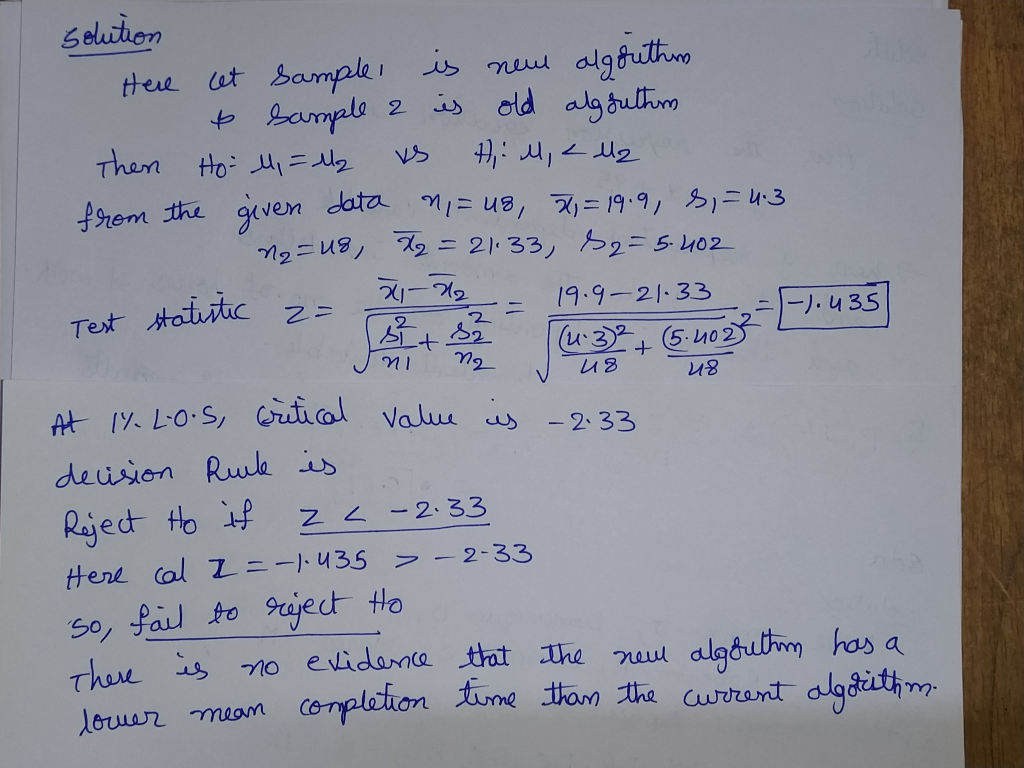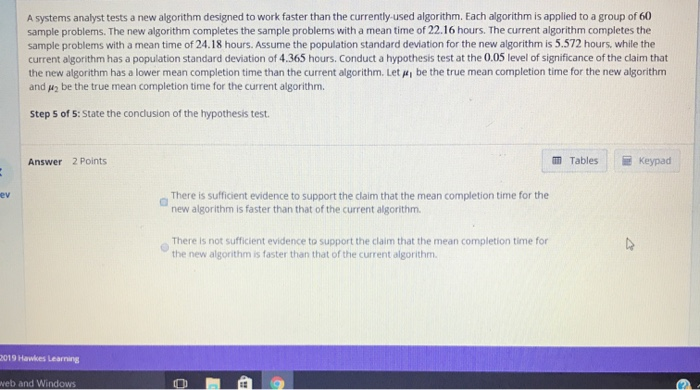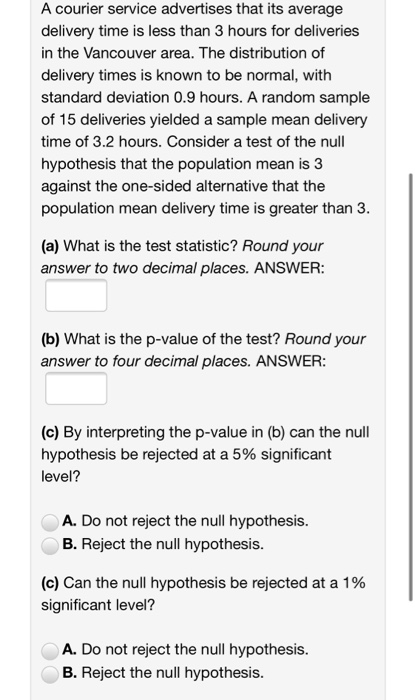Question

# A systems analyst tests a new algorithm designed to work faster than the currently-used algorithm. Each...

A systems analyst tests a new algorithm designed to work faster than the currently-used algorithm. Each algorithm is applied to a group of 48 sample problems. The new algorithm completes the sample problems with a mean time of 19.90 hours. The current algorithm completes the sample problems with a mean time of 21.33 hours. The standard deviation is found to be 4.320 hours for the new algorithm, and 5.402 hours for the current algorithm. Conduct a hypothesis test at the 0.01 level of significance of the claim that the new algorithm has a lower mean completion time than the current algorithm. Let μ1 be the true mean completion time for the new algorithm and μ2 be the true mean completion time for the current algorithm.

Step 1 of 4:

State the null and alternative hypotheses for the test.

H0: ?1 (blank) ?2

Ha: ?1 (blank) ?2

Step 2 of 4:

Compute the value of the test statistic. Round your answer to two decimal places.

Step 3 of 4:

Determine the decision rule for rejecting the null hypothesis H0. Round the numerical portion of your answer to three decimal places.

Reject H0 if (blank) (blank) (blank) [NOTE: First blank choices: z or | z | Second blank choices: > or < Third blank: Round answer to 3 decimal places]

Step 4 of 4: Multiple Choice

a) Reject Null Hypothesis

b) Fail to Reject Null Hypothesis#### Earn Coins

Coins can be redeemed for fabulous gifts.

Similar Homework Help Questions
• ### A systems analyst tests a new algorithm designed to work faster than the currently-used algorithm. Each...

A systems analyst tests a new algorithm designed to work faster than the currently-used algorithm. Each algorithm is applied to a group of 48 sample problems. The new algorithm completes the sample problems with a mean time of 19.90 hours. The current algorithm completes the sample problems with a mean time of 21.33 hours. The standard deviation is found to be 4.320 hours for the new algorithm, and 5.402 hours for the current algorithm. Conduct a hypothesis test at the...

• ### A systems analyst tests a new algorithm designed to work faster than the currently-used algorithm. Each...

A systems analyst tests a new algorithm designed to work faster than the currently-used algorithm. Each algorithm is applied to a group of 3333 sample problems. The new algorithm completes the sample problems with a mean time of 24.0524.05 hours. The current algorithm completes the sample problems with a mean time of 27.0127.01 hours. Assume the population standard deviation for the new algorithm is 5.3295.329 hours, while the current algorithm has a population standard deviation of 3.0783.078 hours. Conduct a...

• ### A systems analyst tests a new algorithm designed to work faster than the currently-used algorithm. Each algorithm is applied to a group of 47sample problems. The new algorithm completes the sample pro...

A systems analyst tests a new algorithm designed to work faster than the currently-used algorithm. Each algorithm is applied to a group of 47sample problems. The new algorithm completes the sample problems with a mean time of 14.75 hours. The current algorithm completes the sample problems with a mean time of 16.77 hours. Assume the population standard deviation for the new algorithm is 3.956 hours, while the current algorithm has a population standard deviation of 5.135 hours. Conduct a hypothesis...

• ### A systems analyst tests a new algorithm designed to work faster than the currently used algorithm....A systems analyst tests a new algorithm designed to work faster than the currently used algorithm. Each algorithm is applied to a group of 60 sample problems. The new algorithm completes the sample problems with a mean time of 22.16 hours. The current algorithm completes the sample problems with a mean time of 24.18 hours. Assume the population standard deviation for the new algorithm is 5.572 hours, while the current algorithm has a population standard deviation of 4.365 hours. Conduct...

Using traditional methods it takes 11.3 hours to receive a basic flying license. A new license training method using Computer Aided Instruction (CAI) has been proposed. A researcher used the technique on 20 students and observed that they had a mean of 11.6 hours with a standard deviation of 1.1. Is there evidence at the 0.05 level that the technique performs differently than the traditional method? Assume the population distribution is approximately normal. Step 1 of 5: State the null...

• ### In order for a new drug to be sold on the market, the variance of the...

In order for a new drug to be sold on the market, the variance of the active ingredient in each dose should be 0.02mg. A random sample of 25 tablets with a dosage strength of 48.42⁢mg is taken. The variance of the active ingredient from this sample is found to be 0.0048. Does the data suggests at α=0.01 that the variance of the drug in the tablets is less than the desired amount? Assume the population is normally distributed. Step...

• ### A courier service advertises that its average delivery time is less than 3 hours for deliveries...A courier service advertises that its average delivery time is less than 3 hours for deliveries in the Vancouver area. The distribution of delivery times is known to be normal, with standard deviation 0.9 hours. A random sample of 15 deliveries yielded a sample mean delivery time of 3.2 hours. Consider a test of the null hypothesis that the population mean is 3 against the one-sided alternative that the population mean delivery time is greater than 3. (a) What is...

• ### The makers of a child's swing set claim that the average assembly time is less than...

The makers of a child's swing set claim that the average assembly time is less than 2 hours. A sample of 35 assembly times (in hours) for this swing set is given in the table below. Test their claim at the 0.10 significance level. (a) What type of test is this? This is a two-tailed test. This is a right-tailed test.     This is a left-tailed test. (b) What is the test statistic? Round your answer to 2 decimal places. tx...

Using traditional methods it takes 106 hours to receive an advanced flying license. A new training technique using Computer Aided Instruction (CAI) has been proposed. A researcher used the technique on 40 students and observed that they had a mean of 105 hours. Assume the population standard deviation is known to be 4. Is there evidence at the 0.02 level that the technique reduces the training time? Step 1 of 6: State the null and alternative hypotheses. Step 2 of...

• ### The director of research and development is testing a new medicine. She wants to know if...

The director of research and development is testing a new medicine. She wants to know if there is evidence at the 0.1 level that the medicine relieves pain in more than 358 seconds. For a sample of 62 patients, the mean time in which the medicine relieved pain was 366 seconds. Assume the population standard deviation is 21 . Step 1 of 6: State the null and alternative hypotheses. Step 2 of 6: Find the value of the test statistic....